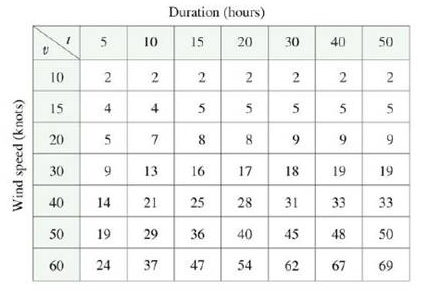Chapter 14.3, Problem 4E

Chapter
Section
Textbook Problem

The wave heights h in the open sea depend on the speed v of the wind and the length of time t that the wind has been blowing at that speed. Values of the function h = f(v, t) are recorded in feet in the following table.(a) What are the meanings of the partial derivatives ∂h/∂v and ∂h/∂t?(b) Estimate the values of fv(40, 15) and ft(40, 15). What are the practical interpretations of these values?(c) What appears to be the value of the following limit? lim t → ∞ ∂ h ∂ t

(a)

To determine

To explain: The meaning of the first order partial derivatives hv and ht where h=f(v,t) .

Explanation

The given function is h=f(v,t) , where h is the wave height, t is the length of time and v is the wind speed.

The partial derivative h

(b)

To determine

To estimate: The values of fv(40,15) and ft(40,15) from the given table and interpret the values.

(c)

To determine

To find: The value of limtht .

Still sussing out bartleby?

Check out a sample textbook solution.

See a sample solution

The Solution to Your Study Problems

Bartleby provides explanations to thousands of textbook problems written by our experts, many with advanced degrees!

Get Started

Evaluate the integral. xx27dx

Calculus (MindTap Course List)

In Exercises 4144, determine whether the statement is true or false. 41. 3 20

Applied Calculus for the Managerial, Life, and Social Sciences: A Brief Approach

Evaluate the integrals in Problems 1-11.

Mathematical Applications for the Management, Life, and Social Sciences

What is the integrating factor for xy′ + 6x2y = 10 − x3?

Study Guide for Stewart's Single Variable Calculus: Early Transcendentals, 8th

0 1 ∞

Study Guide for Stewart's Multivariable Calculus, 8th